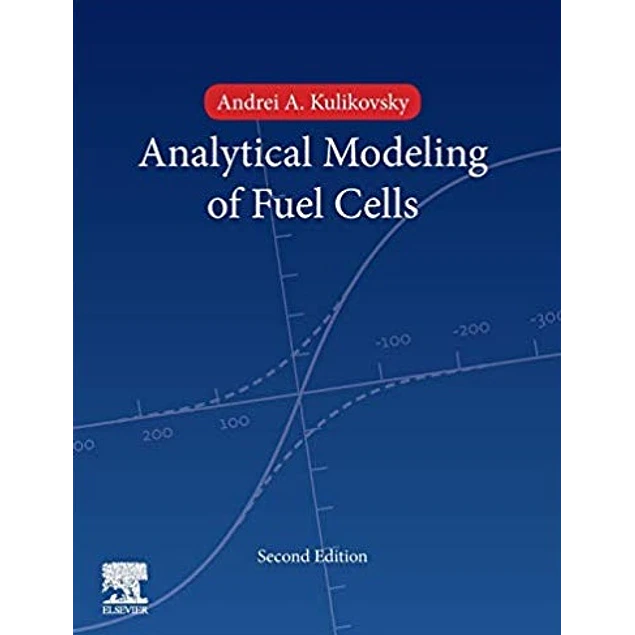## Analytical Modelling of Fuel Cells

10.00\$# Analytical Modelling of Fuel Cells

10.00\$

2nd 2019 Edition

by Andrei A. Kulikovsky (Author)

Analytical Modelling of Fuel Cells, Second Edition, is devoted to the analytical models that help us understand the mechanisms of cell operation. The book contains equations for the rapid evaluation of various aspects of fuel cell performance, including cell potential, rate of electrochemical reactions, rate of transport processes in the cell, and temperature fields in the cell, etc. Furthermore, the book discusses how to develop simple physics-based analytical models. A new chapter is devoted to analytical models of PEM fuel cell impedance, a technique that exhibits explosive growth potential. Finally, the book contains Maple worksheets implementing some of the models discussed.

• Includes simple physics-based equations for the fuel cell polarization curve
• Provides analytical solutions for fuel cell impedance
• Includes simple equations for calculation of temperature shapes in fuel cells
• Introduces physical descriptions of the basic transport and kinetic phenomena in fuel cells of various types
Year:
2019
Pages:
373
Language:
English
Format:
PDf
Size:
9.5 MB
ISBN-10:
444642226
ISBN-13:
978-0444642226
ASIN:
B07RJD3Q1F
##### Contact
•••••